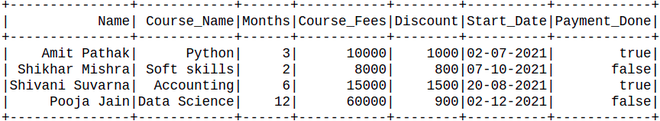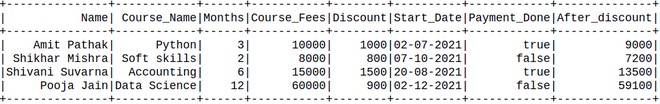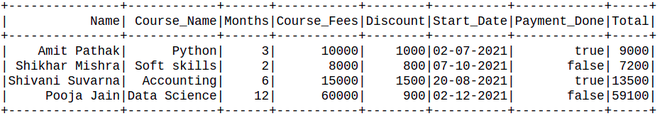Open in App
Not now

# PySpark dataframe add column based on other columns

• Difficulty Level : Medium
• Last Updated : 23 Aug, 2021

In this article, we are going to see how to add columns based on another column to the Pyspark Dataframe.

Creating Dataframe for demonstration:

Here we are going to create a dataframe from a list of the given dataset.

## Python3

 `# Create a spark session``from` `pyspark.sql ``import` `SparkSession``spark ``=` `SparkSession.builder.appName(``'SparkExamples'``).getOrCreate()`` ` `# Create a spark dataframe``columns ``=` `[``"Name"``, ``"Course_Name"``,``           ``"Months"``,``           ``"Course_Fees"``, ``"Discount"``,``           ``"Start_Date"``, ``"Payment_Done"``]``data ``=` `[``    ``(``"Amit Pathak"``, ``"Python"``, ``3``, ``10000``, ``1000``,``     ``"02-07-2021"``, ``True``),``    ``(``"Shikhar Mishra"``, ``"Soft skills"``, ``2``,``     ``8000``, ``800``, ``"07-10-2021"``, ``False``),``    ``(``"Shivani Suvarna"``, ``"Accounting"``, ``6``,``     ``15000``, ``1500``, ``"20-08-2021"``, ``True``),``    ``(``"Pooja Jain"``, ``"Data Science"``, ``12``,``     ``60000``, ``900``, ``"02-12-2021"``, ``False``),``]`` ` `df ``=` `spark.createDataFrame(data).toDF(``*``columns)`` ` `# View the dataframe``df.show()`

Output:## Method 1: Using withColumns()

It is used to change the value, convert the datatype of an existing column, create a new column, and many more.

Syntax: df.withColumn(colName, col)

Returns: A new :class:`DataFrame` by adding a column or replacing the existing column that has the same name.

## Python3

 `new_df ``=` `df.withColumn(``'After_discount'``,``                       ``df.Course_Fees ``-` `df.Discount)``new_df.show()`

Output:## Method 2: Using SQL query

Here we will use SQL query inside the Pyspark, We will create a temp view of the table with the help of createTempView() and the life of this temp is up to the life of the sparkSession. registerTempTable() will create the temp table if it is not available or if it is available then replace it.

Then after creating the table select the table by SQL clause which will take all the values as a string.

## Python3

 `df.registerTempTable(``'table'``)``newDF ``=` `spark.sql(``'select *, Course_Fees - Discount as Total from table'``)``newDF.show()`

Output:## Method 3: Using UDF

In this method, we will define the user define a function that will take two parameters and return the total price. This function allows us to create a new function as per our requirements.

Now we define the data type of the UDF function and create the functions which will return the values which is the sum of all values in the row.

## Python3

 `# import the functions as F from pyspark.sql``import` `pyspark.sql.functions as F``from` `pyspark.sql.types ``import` `IntegerType`` ` `# define the sum_col``def` `Total(Course_Fees, Discount):``    ``res ``=` `Course_Fees ``-` `Discount``    ``return` `res`` ` `# integer datatype is defined``new_f ``=` `F.udf(Total, IntegerType())`` ` `# calling and creating the new``# col as udf_method_sum``new_df ``=` `df.withColumn(``  ``"Total_price"``, new_f(``"Course_Fees"``, ``"Discount"``))`` ` `# Showing the Dataframe``new_df.show()`

Output:My Personal Notes arrow_drop_up